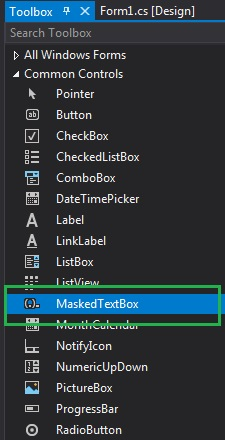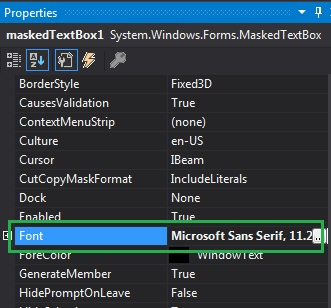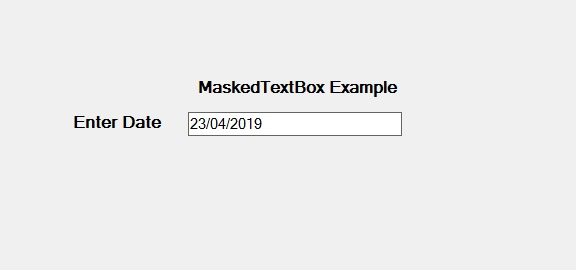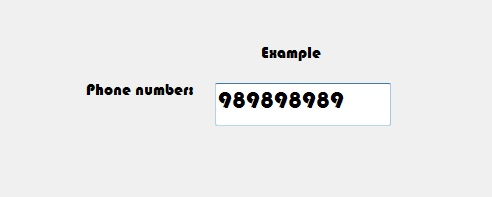Skip to content
Related Articles
How to set the Font of the MaskedTextBox in C#?
• Last Updated : 07 Aug, 2019

In C#, MaskedTextBox control gives a validation procedure for the user input on the form like date, phone numbers, etc. Or in other words, it is used to provide a mask which differentiates between proper and improper user input. In MaskedTextBox control, you can set the font of the content present in the MaskedTextBox using Font Property. This property is ambient property. You can set this property in two different ways:

1. Design-Time: It is the easiest way to set the font of the MaskedTextBox as shown in the following steps:

• Step 1: Create a windows form as shown in the below image:

Visual Studio -> File -> New -> Project -> WindowsFormApp• Step 2: Next, drag and drop the MaskedTextBox control from the toolbox on the form.• Step 3: After drag and drop you will go to the properties of the MaskedTextBox and set the font of the MaskedTextBox.Output:2. Run-Time: It is a little bit trickier than the above method. In this method, you can set the font of the MaskedTextBox control programmatically with the help of given syntax:

`public virtual System.Drawing.Font Font { get; set; }`

Here, Font represents the font of the content present in the MaskedTextBox. The following steps show how to set the font of the MaskedTextBox dynamically:

• Step 1: Create a MaskedTextBox using the MaskedTextBox() constructor is provided by the MaskedTextBox class.
```// Creating a MaskedTextBox
MaskedTextBox m = new MaskedTextBox();
```
• Step 2: After creating MaskedTextBox, set the Font property of the MaskedTextBox provided by the MaskedTextBox class.
```// Setting the font
m.Font = new Font("Bauhaus 93", 18);
```
• Step 3: And last add this MaskedTextBox control to the form using the following statement:
```// Adding MaskedTextBox control on the form
this.Controls.Add(m);
```

Example:

 `using` `System;``using` `System.Collections.Generic;``using` `System.ComponentModel;``using` `System.Data;``using` `System.Drawing;``using` `System.Linq;``using` `System.Text;``using` `System.Threading.Tasks;``using` `System.Windows.Forms;`` ` `namespace` `WindowsFormsApp36 {`` ` `public` `partial` `class` `Form1 : Form {`` ` `    ``public` `Form1()``    ``{``        ``InitializeComponent();``    ``}`` ` `    ``private` `void` `Form1_Load(``object` `sender, EventArgs e)``    ``{``        ``// Creating and setting the``        ``// properties of the Label``        ``Label l1 = ``new` `Label();``        ``l1.Location = ``new` `Point(413, 98);``        ``l1.Size = ``new` `Size(176, 20);``        ``l1.Text = ``" Example"``;``        ``l1.Font = ``new` `Font(``"Bauhaus 93"``, 12);`` ` `        ``// Adding label on the form``        ``this``.Controls.Add(l1);`` ` `        ``// Creating and setting ``        ``// the properties of Label``        ``Label l2 = ``new` `Label();``        ``l2.Location = ``new` `Point(242, 135);``        ``l2.Size = ``new` `Size(126, 20);``        ``l2.Text = ``"Phone number:"``;``        ``l2.Font = ``new` `Font(``"Bauhaus 93"``, 12);`` ` `        ``// Adding label on the form``        ``this``.Controls.Add(l2);`` ` `        ``// Creating and setting the ``        ``// properties of MaskedTextBox``        ``MaskedTextBox m = ``new` `MaskedTextBox();``        ``m.Location = ``new` `Point(374, 137);``        ``m.Mask = ``"000000000"``;``        ``m.Size = ``new` `Size(176, 20);``        ``m.Name = ``"MyBox"``;``        ``m.Font = ``new` `Font(``"Bauhaus 93"``, 18);`` ` `        ``// Adding MaskedTextBox ``        ``// control on the form``        ``this``.Controls.Add(m);``    ``}``}``}`

Output:My Personal Notes arrow_drop_up